# MAX function in Excel: formula examples to find and highlight highest value

The tutorial explains the MAX function with many formula examples that show how to find highest value in Excel and highlight largest number in your worksheet.

MAX is one of the most straightforward and easy-to-use Excel functions. However, it does have a couple of tricks knowing which will give you a big advantage. Say, how do you use the MAX function with conditions? Or how would you extract the absolute largest value? This tutorial provides more than one solution for these and other related tasks.

## Excel MAX function

The MAX function in Excel returns the highest value in a set of data that you specify.

The syntax is as follows:

MAX(number1, [number2], …)

Where number can be represented by a numeric value, array, named range, a reference to a cell or range containing numbers.

Number1 is required, number2 and subsequent arguments are optional.

The MAX function is available in all versions of Excel for Office 365, Excel 2019, Excel 2016, Excel 2013, Excel 2010, Excel 2007, and lower.

## How to make a MAX formula in Excel

To create a MAX formula in its simplest from, you can type numbers directly in the list of arguments, like this:

`=MAX(1, 2, 3)`

In practice, it's quite a rare case when numbers are "hardcoded". For the most part, you will deal with ranges and cells.

The fastest way to build a Max formula that finds the highest value in a range is this:

1. In a cell, type =MAX(
2. Select a range of numbers using the mouse.
3. Type the closing parenthesis.
4. Press the Enter key to complete your formula.

For example, to work out the largest value in the range A1:A6, the formula would go as follows:

`=MAX(A1:A6)`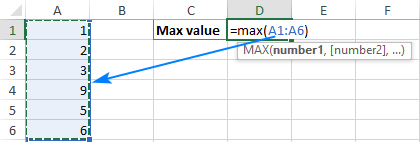If your numbers are in a contiguous row or column (like in this example), you can get Excel to make a Max formula for you automatically. Here's how:

1. Select the cells with your numbers.
2. On the Home tab, in the Formats group, click AutoSum and pick Max from the drop-down list. (Or click AutoSum > Max on the Formulas tab in the Function Library group.)

This will insert a ready-to-use formula in a cell below the selected range, so please make sure there is at least one blank cell underneath the list of numbers that you've selected: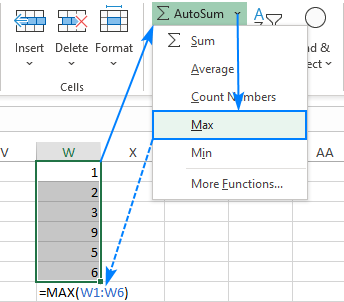### 5 things to know about MAX function

To successfully use Max formulas your worksheets, please remember these simple facts:

1. In the current versions of Excel, a MAX formula can accept up to 255 arguments.
2. If the arguments do not contain a single number, the MAX function returns zero.
3. If the arguments contain one or more error values, an error is returned.
4. Empty cells are ignored.
5. Logical values and text representations of numbers supplied directly in the list of arguments are processed (TRUE evaluates as 1, FALSE evaluates as 0). In references, logical and text values are ignored.

## How to use MAX function in Excel – formula examples

Below you will find a few typical uses of the Excel MAX function. In many cases, there are a few different solutions for the same task, so I encourage you to test all the formulas to choose the one best suited for your data type.

### How to find max value in a group

To extract the largest number in a group of numbers, supply that group to the MAX function as a range reference. A range can contain as many rows and columns as you desire. For example, to get the highest value in the range C2:E7, use this simple formula:

`=MAX(C2:E7)`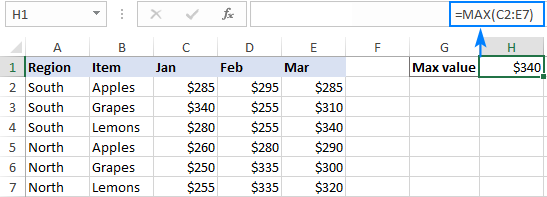### Find highest value in non-adjacent cells or ranges

To make a MAX formula for non-contiguous cells and ranges, you need to include a reference to each individual cell and/or range. The following steps will help you to do that quickly and flawlessly:

1. Start typing a Max formula in a cell.
2. After you've typed the opening parenthesis, hold down the Ctrl key and select the cells and ranges in the sheet.
3. After selecting the last item, release Ctrl and type the closing parenthesis.
4. Press Enter.

Excel will use an appropriate syntax automatically, and you will get a formula similar to this:

`=MAX(C5:E5, C9:E9)`

As shown in the screenshot below, the formula returns the maximum sub-total value from rows 5 and 9: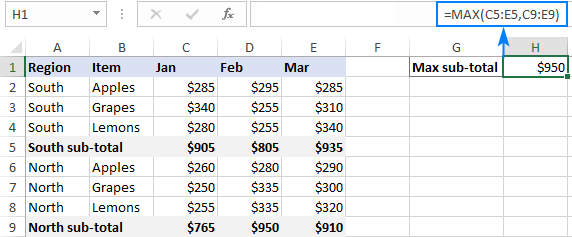### How to get max (latest) date in Excel

In the internal Excel system, dates are nothing else but serial numbers, so the MAX function handles them without a hitch.

For instance, to find the latest delivery date in C2:C7, make a usual Max formula that you'd use for numbers:

`=MAX(C2:C7)`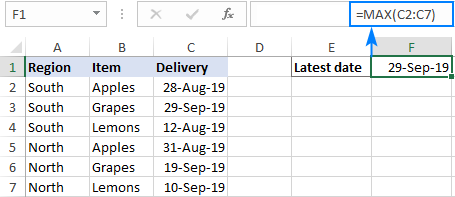### MAX function in Excel with conditions

When you wish to get the maximum value based on conditions, there are several formulas for you to choose from. To make sure that all the formulas return the identical result, we will test them on the same set of data.

The task: With the items listed in B2:B15 and sales figures in C2:C15, we aim to find the highest sale for a specific item input in F1 (please see the screenshot at the end of this section).

#### Excel MAX IF formula

If you a looking for a formula that works in all versions of Excel 2000 through Excel 2019, use the IF function to test the condition, and then pass the resulting array to the MAX function:

`=MAX(IF(B2:B15=F1, C2:C15))`

For the formula to work, it must press Ctrl + Shift + Enter simultaneously to enter it as an array formula. If all done correctly, Excel will enclose your formula in {curly braces}, which is a visual indication of an array formula.

It is also possible to evaluate several conditions in a single formula, and the following tutorial shows how: MAX IF with multiple conditions.

#### Non-array MAX IF formula

If you don't like using array formulas in your worksheets, then combine MAX with the SUMPRODUCT function that processes arrays natively:

`=SUMPRODUCT(MAX((B2:B15=F1)*(C2:C15)))`

#### MAXIFS function

In Excel 2019 and Excel for Office 365, there is a special function named MAXIFS, which is designed to find the highest value with up to 126 criteria.

In our case, there is just one condition, so the formula is as simple as:

`=MAXIFS(C2:C15, B2:B15, F1)`

For the detailed explanation of the syntax, please see Excel MAXIFS with formula examples.

The below screenshot shows all 3 formulas in action: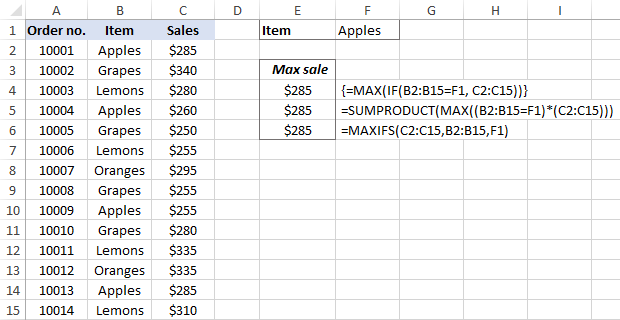### Get max value ignoring zeros

This is, in fact, a variation of conditional MAX discussed in the previous example. To exclude zeros, use the "not equal to" logical operator and put the expression "<>0" in either the criteria of MAXIFS or the logical test of MAX IF.

As you understand, testing this condition only makes sense in case of negative numbers. With positive numbers, this check is superfluous because any positive number is greater than zero.

To give it a try, let's find the lowest discount in the range C2:C7. As all the discounts are represented by negative numbers, the smallest discount is actually the largest value.

#### MAX IF

Be sure to press Ctrl + Shift + Enter to correctly complete this array formula:

`=MAX(IF(C2:C7<>0, C2:C7))`

#### MAXIFS

It's a regular formula, and a usual Enter keystroke will suffice.

`=MAXIFS(C2:C7,C2:C7,"<>0")`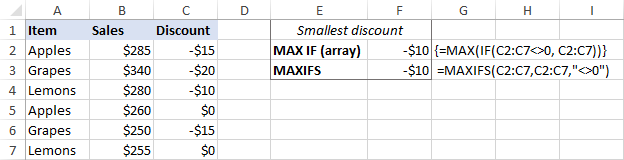### Find highest value ignoring errors

When you work with a large amount of data driven by various formulas, chances are that some of your formulas will result in errors, which will cause a MAX formula to return an error too.

As a workaround, you can use MAX IF together with ISERROR. Given that you are searching in the range A1:B5, the formula takes this shape:

`=MAX(IF(ISERROR(A1:B5)), "", A1:B5))`

To simplify the formula, use the IFERROR function instead of the IF ISERROR combination. This will also make the logic a bit more obvious – if there's an error in A1:B5, replace it with an empty string (''), and then get the maximum value in the range:

`=MAX(IFERROR(A1:B5, ""))`

A fly in the ointment is that you need to remember to press Ctrl + Shift + Enter because this only works as an array formula.

In Excel 2019 and Excel for Office 356, the MAXIFS function can be a solution, provided that your data set contains at least one positive number or zero value:

`=MAXIFS(A1:B5,A1:B5,">=0")`

Since the formula searches for the highest value with the condition "greater than or equal to 0", it won't work for a data set consisting of solely negative numbers.

All these limitations are not good, and we are evidently in need of a better solution. The AGGREGATE function, which can perform a number of operations and ignore error values, fits perfectly:

`=AGGREGATE(4, 6, A1:B5)`

The number 4 in the 1st argument indicates the MAX function, the number 6 in the 2nd argument is the "ignore errors" option, and A1:B5 is your target range.

Under perfect circumstances, all three formulas will return the same result: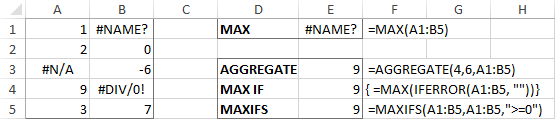### How to find absolute max value in Excel

When working with a range of positive and negative numbers, sometimes you may wish to find the largest absolute value regardless of the sign.

The first idea that comes to mind is to get the absolutes values of all numbers in the range by using the ABS function and feed those to MAX:

{=MAX(ABS(range))}

This is an array formula, so don't forget to confirm it with the Ctrl + Shift + Enter shortcut. Another caveat is that it only works with numbers and results in an error in case of non-numeric data.

Not happy with this formula? Then let us build something more viable :)

What if we find the minimum value, reverse or ignore its sign, and then evaluate along with all other numbers? Yep, that will work perfectly as a normal formula. As an extra bonus, it handles text entries and errors just fine:

With the source numbers in A1:B5, the formulas go as follows.

Array formula (completed with Ctrl + Shift + Enter):

`=MAX(ABS(A1:B5))`

Regular formula (completed with Enter):

`=MAX(MAX(A1:B5), -MIN(A1:B5))`

or

`=MAX(MAX(A1:B5), ABS(MIN(A1:B5)))`

The below screenshot shows the results: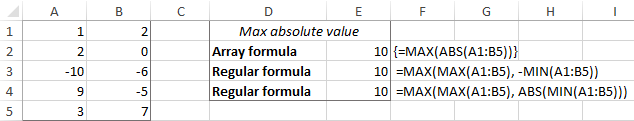#### Return the maximum absolute value preserving the sign

In some situations, you may have a need to find the largest absolute value but return the number with its original sign, not the absolute value.

Assuming the numbers are in cells A1:B5, here's the formula to use:

`=IF(ABS(MAX(A1:B5))>ABS(MIN(A1:B5)), MAX(A1:B5), MIN(A1:B5))`

Complex at first sight, the logic is quite easy to follow. First, you find the largest and smallest numbers in the range and compare their absolute values. If the absolute max value is greater than the absolute min value, the maximum number is returned, otherwise – the minimum number. Because the formula returns the original and not absolute value, it keeps the sign information: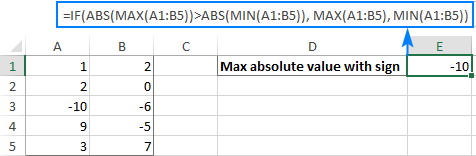## How to highlight max value in Excel

In situation when you want to identify the largest number in the original data set, the fastest way is to highlight it with Excel conditional formatting. The below examples will walk you through two different scenarios.

### Highlight highest number in a range

Microsoft Excel has a predefined rule to format top ranked values, which suits our needs perfectly. Here are the steps to apply it:

1. Select your range of numbers (C2:C7 in our case).
2. On the Home tab, in the Styles group, click Conditional formatting > New Rule.
3. In the New Formatting Rule dialog box, choose Format only top or bottom ranked values.
4. In the lower pane, pick Top from the drop-down list and type 1 in the box next to it (meaning you want to highlight just one cell containing the largest value).
5. Click the Format button and select the desired format.
6. Click OK twice to close both windows.

Done! The highest value in the selected range is automatically highlighted. If there is more than one max value (duplicates), Excel will highlight them all: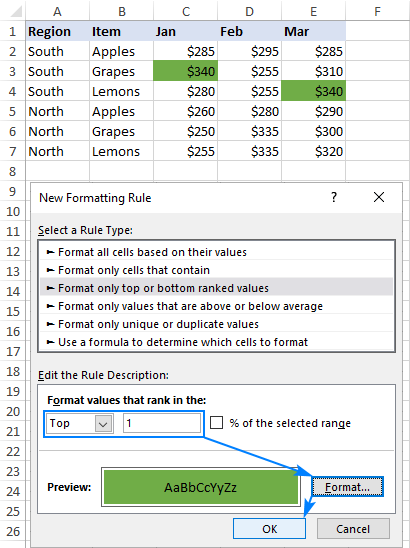### Highlight max value in each row

Since there is no built-in rule to make the highest value stand out from each row, you will have to configure your own one based on a MAX formula. Here's how:

1. Select all the rows in which you want to highlight max values (C2:C7 in this example).
2. On the Home tab, in the Styles group, click New Rule > Use a formula to determine which cells to format.
3. In the Format values where this formula is true box, enter this formula:

`=C2=MAX(\$C2:\$E2)`

Where C2 is the leftmost cell and \$C2:\$E2 is the first row range. For the rule to work, be sure to lock the column coordinates in the range with the \$ sign.

4. Click the Format button and choose the format you want.
5. Click OK twice.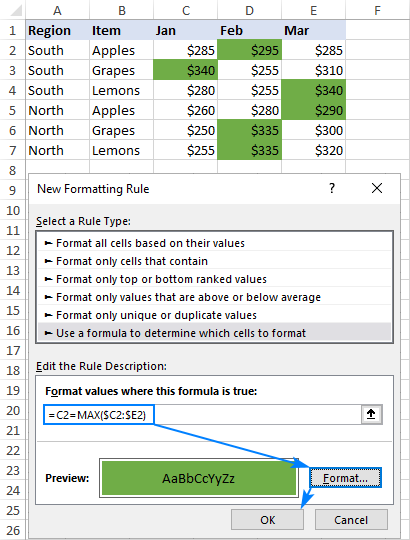Tip. In a similar manner, you can highlight the highest value in each column. The steps are exactly the same, except that you write a formula for the first column range and lock the row coordinates: `=C2=MAX(C\$2:C\$7)`

## Excel MAX function not working

MAX is one of the most straightforward Excel functions to use. If against all expectations it does not work right, it's most likely to be one of the following issues.

### MAX formula returns zero

If a normal MAX formula returns 0 even though there are higher numbers in the specified range, chances are those numbers are formatted as text. It's especially the case when you run the MAX function on data driven by other formulas. You can check this by using the ISNUMBER function, for example:

`=ISNUMBER(A1)`

If the above formula returns FALSE, the value in A1 is not numeric. Meaning, you should troubleshoot the original data, not a MAX formula.

### MAX formula returns #N/A, #VALUE or other error

Please check the referenced cells carefully. If any of the referenced cells contains an error, a MAX formula will result in the same error. To bypass this, see how to get the max value ignoring all errors.

That's how to find max value in Excel. I thank you for reading and hope to see you on our blog soon!

## You may also be interested in

1. Thx..could you give the formula to return the region after finding the Max from the three months?
Regards,
Larry

• Hi! If I understand correctly, use INDEX MATCH functions to find the row with the maximum value.
For example,

=INDEX(A1:A5,MIN(IF(C2:F5=MAX(C2:F5),ROW(A2:A5))))

2. This is very great. Please I want to find the MAX but to replace the value with the name how do I do that?

• Hi! You cannot replace a number in a cell with a name using an Excel formula. If I have misunderstood, please explain your question in more detail.

3. This blog is the most comprehensive but I just need one more step.
My set of data is a measure of both positive and negative deviation from 0 with so called 'error' cells too. I'm looking for formula to give me maximum value from zero including its sign.
It looks like I could do with a combination of Index / match / aggregate. Data example is
Column A : #N/A, #N/A, 6, 5, -2, 0, 0, -5, -3, -4, #N/A, #N/A, -10, -15, -12, 0, 11
The formula result would then be -15